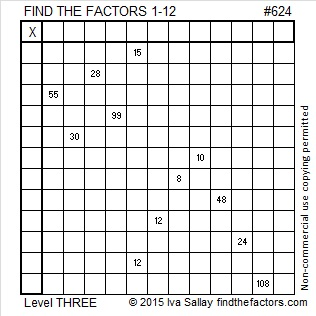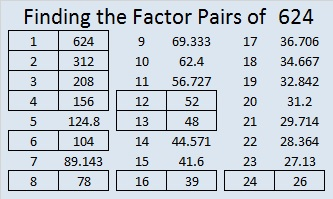# 624 and Level 3

Since 624 is one less than 625 which is 25 squared, it should be obvious that 24 x 26 = 624.

624 is the hypotenuse of the Pythagorean triple 240-576-624. What is the greatest common factor of those three numbers?

624 is the sum of consecutive odd numbers and twin primes 311 and 313.Print the puzzles or type the solution on this excel file: 12 Factors 2015-09-21

—————————————————————————————————

• 624 is a composite number.
• Prime factorization: 624 = 2 x 2 x 2 x 2 x 3 x 13, which can be written 624 = (2^4) x 3 x 13
• The exponents in the prime factorization are 4, 1 and 1. Adding one to each and multiplying we get (4 + 1)(1 + 1)(1 + 1) = 5 x 2 x 2 = 20. Therefore 624 has exactly 20 factors.
• Factors of 624: 1, 2, 3, 4, 6, 8, 12, 13, 16, 24, 26, 39, 48, 52, 78, 104, 156, 208, 312, 624
• Factor pairs: 624 = 1 x 624, 2 x 312, 3 x 208, 4 x 156, 6 x 104, 8 x 78, 12 x 52, 13 x 48, 16 x 39 or 24 x 26
• Taking the factor pair with the largest square number factor, we get √624 = (√16)(√39) = 4√39 ≈ 24.97999199.—————————————————————————————————

A Logical Approach to solve a FIND THE FACTORS puzzle: Find the column or row with two clues and find their common factor. (None of the factors are greater than 12.)  Write the corresponding factors in the factor column (1st column) and factor row (top row).  Because this is a level three puzzle, you have now written a factor at the top of the factor column. Continue to work from the top of the factor column to the bottom, finding factors and filling in the factor column and the factor row one cell at a time as you go.